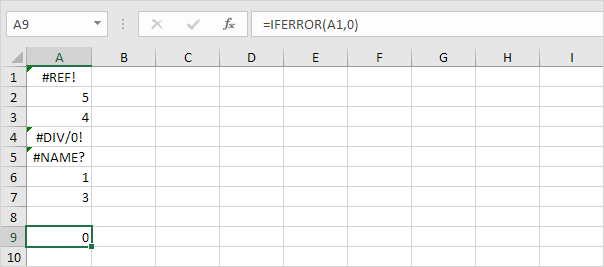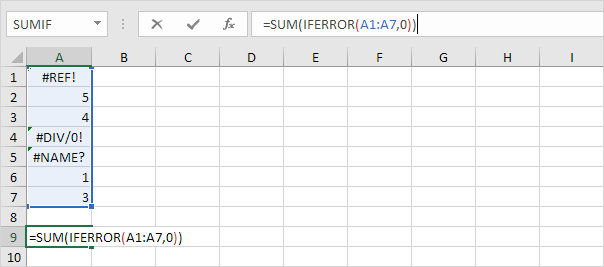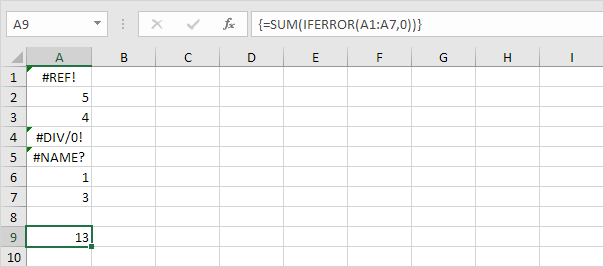## Excel Office

Excel How Tos, Tutorials, Tips & Tricks, Shortcuts

# How to Sum Range with Errors in Excel

This example shows you how to create an array formula that sums a range with errors. You can also use the AGGREGATE function to sum a range with errors.

1. We use the IFERROR function to check for an error.Explanation: the IFERROR function returns 0, if an error is found. If not, it returns the value of the cell.

Worked Example:   Lookup and sum column in Excel

2. To sum the range with errors (don’t be overwhelmed), we add the SUM function and replace A1 with A1:A7.

Worked Example:   Sum range with INDEX in Excel3. Finish by pressing CTRL + SHIFT + ENTER.Note: The formula bar indicates that this is an array formula by enclosing it in curly braces {}. Do not type these yourself. They will disappear when you edit the formula.

Worked Example:   Common Errors in Excel

Explanation: The range (array constant) created by the IFERROR function is stored in Excel’s memory, not in a range. The array constant looks as follows:

{0;5;4;0;0;1;3}

This array constant is used as an argument for the SUM function, giving a result of 13.